# SQL Query to Find all the Students with Marks Greater than Average Marks

• Last Updated : 19 Apr, 2021

Query in SQL is like a statement that performs a task. Here, we need to write a query to find all the students whose marks are greater than the average marks of students.

We will first create a database named “geeks” then we will create a table “Students” in that database.

### Create a Database:

We can create a Database using the command:

`Syntax: CREATE DATABASE DATABASE_NAME;`

So let’s create a geeks database as shown below:

`CREATE DATABASE geeks;`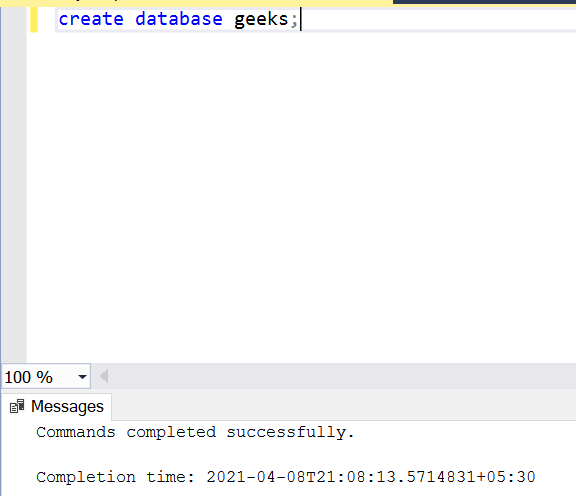### Using Database:

Use the below command to use the geeks database:

`USE geeks;`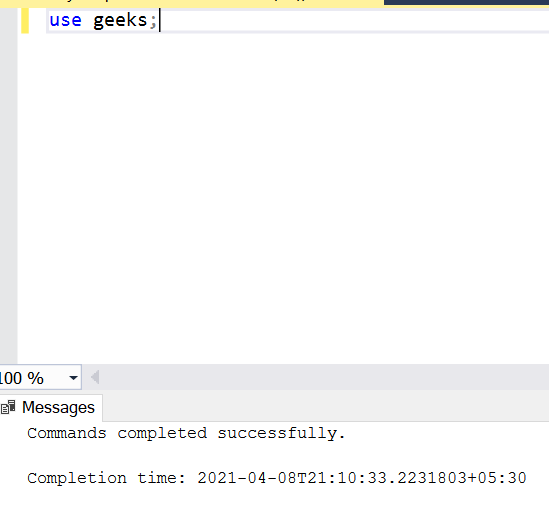To add a table into the database we use the below command:

`Syntax: CREATE TABLE table_name (Attribute_name datatype...);`

So, let’s create a students table within the geeks database as shown below:

```CREATE TABLE Students(
Id int,
Name varchar(20),
TotalMarks int);```

To see the description of the table:

`EXEC sp_columns Students;`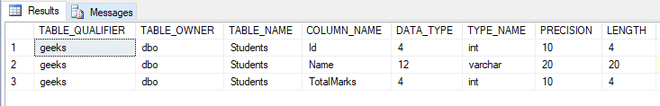### Inserting values into Tables:

For inserting records into the table we can use the below command:

```Syntax: INSERT INTO table_name(column1,
column2,
column 3,.....)
VALUES( value1,
value2,
value3,.....);```

So let’s add some records to the students table:

```INSERT INTO Students VALUES (1,'Neha',90);
INSERT INTO Students VALUES (2,'Sahil',50);
INSERT INTO Students VALUES (3,'Rohan',70);
INSERT INTO Students VALUES (4,'Ankita',80);
INSERT INTO Students VALUES (5,'Rahul',65);
INSERT INTO Students VALUES (6,'Swati',55);
INSERT INTO Students VALUES (7,'Alka',75);```Now let us print the data available in the table using the SELECT statement as shown below:

`SELECT * FROM department;`

Note: Here * represents all. If we execute this query, the entire table will be displayed.

Output :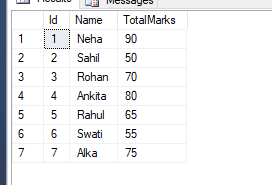### The queryfor the data:

Use the below syntax for querying for all students with greater marks than the average of the class:

```Syntax:
SELECT column1 FROM table_name
WHERE column2 > (SELECT AVG(
column2)
FROM table_name);```

Now use the above syntax to make the query on our students table as shown below:

`SELECT Name FROM Students WHERE TotalMarks > (SELECT AVG(TotalMarks) FROM Students);`

Output: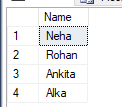My Personal Notes arrow_drop_up